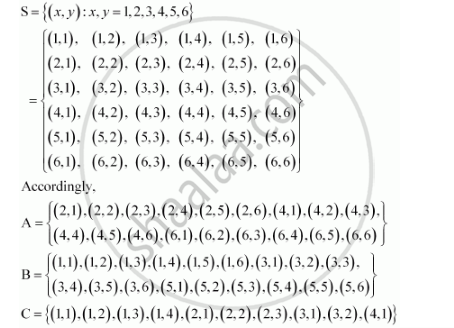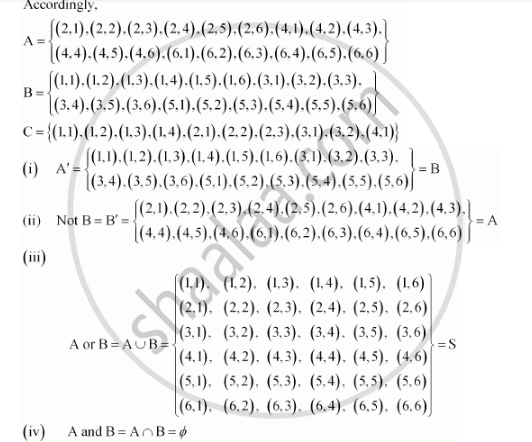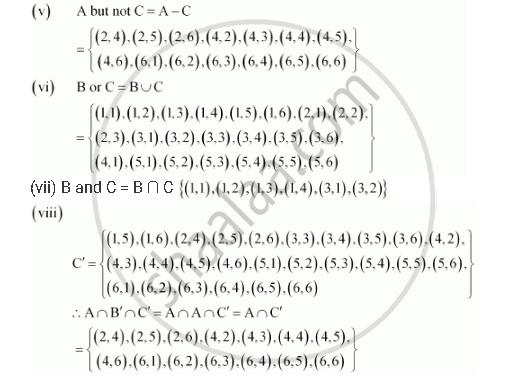CBSE (Arts) Class 11CBSE
Share

# Two Dice Are Thrown. the Events A, B and C Are as Follows: Describe the Events - CBSE (Arts) Class 11 - Mathematics

ConceptEvent Types of Events

#### Question

Two dice are thrown. The events A, B and C are as follows:

A: getting an even number on the first die.

B: getting an odd number on the first die.

C: getting the sum of the numbers on the dice ≤ 5

Describe the events

(i) A'

(ii) not B

(iii) A or B

(iv) A and B

(v) A but not C

(vi) B or C

(vii) B and C

(viii) A ∩ B' ∩ C'

#### Solution

When two dice are thrown, the sample space is given byIs there an error in this question or solution?

#### Video TutorialsVIEW ALL 

Solution Two Dice Are Thrown. the Events A, B and C Are as Follows: Describe the Events Concept: Event - Types of Events.
S